This site uses cookies. By continuing to browse the ConceptDraw site you are agreeing to our Use of Site Cookies.
ConceptDraw Samples | Computer and networks — IVR network

# Interactive Voice Response Diagrams

Samples of interactive voice response (IVR) network diagrams are created using ConceptDraw DIAGRAM diagramming and vector drawing software enhanced with solution Computer and Networks from ConceptDraw Solution Park.

ConceptDraw DIAGRAM provides export of vector graphic multipage documents into multiple file formats: vector graphics (SVG, EMF, EPS), bitmap graphics (PNG, JPEG, GIF, BMP, TIFF), web documents (HTML, PDF), PowerPoint presentations (PPT), Adobe Flash (SWF).

## Tutorials and Solutions:

Video Tutorials: ConceptDraw Solution Park

## Sample 1: IVR Balance Recharge Diagram

Interactive Voice Response Diagrams sample: IVR Balance Recharge Diagram.

This example is created using ConceptDraw DIAGRAM diagramming and vector drawing software enhanced with Interactive Voice Response Diagrams solution from ConceptDraw Solution Park.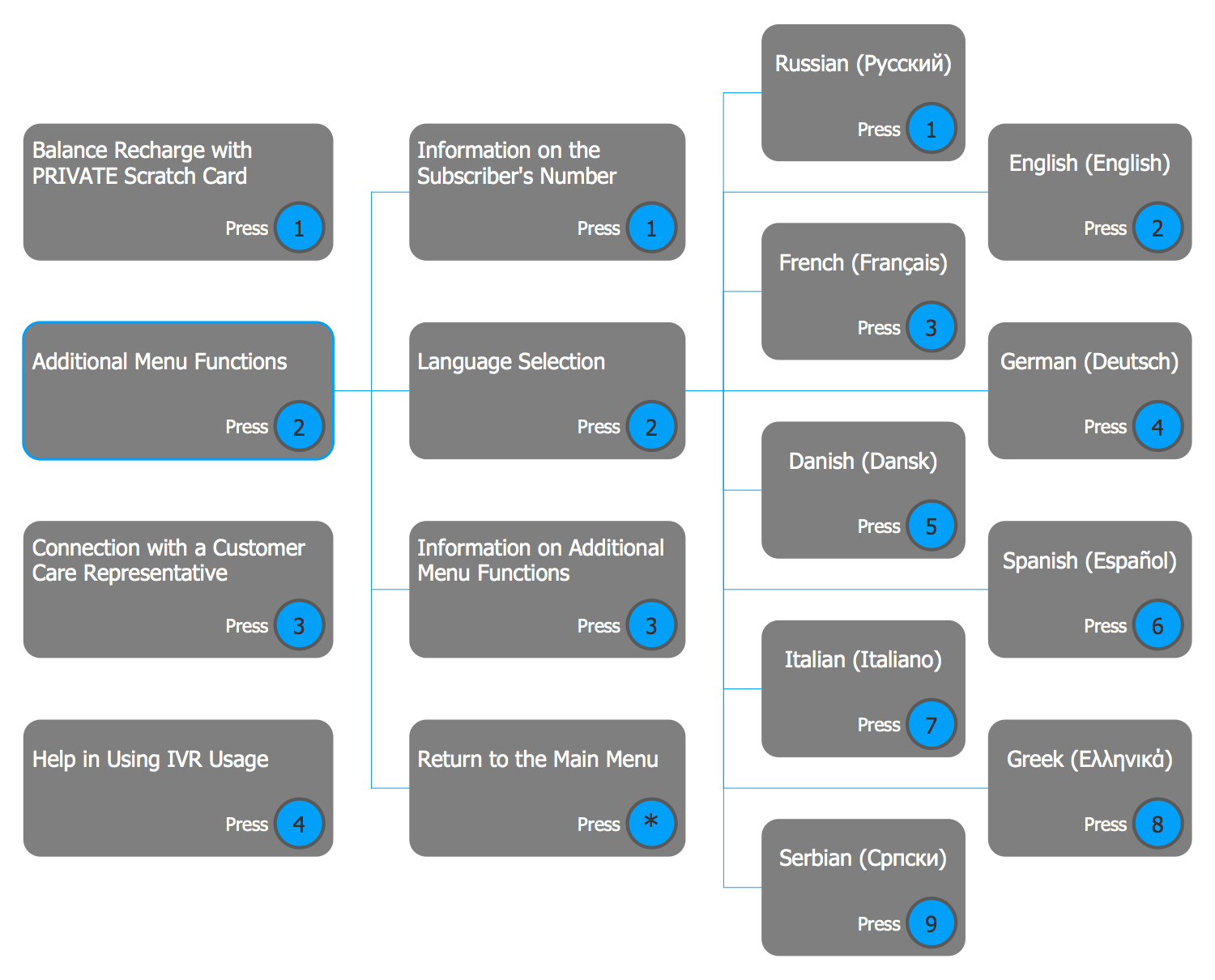## Sample 2: IVR Flowchart

Interactive Voice Response Diagrams sample: IVR Flowchart.

This example is created using ConceptDraw DIAGRAM diagramming and vector drawing software enhanced with Interactive Voice Response Diagrams solution from ConceptDraw Solution Park.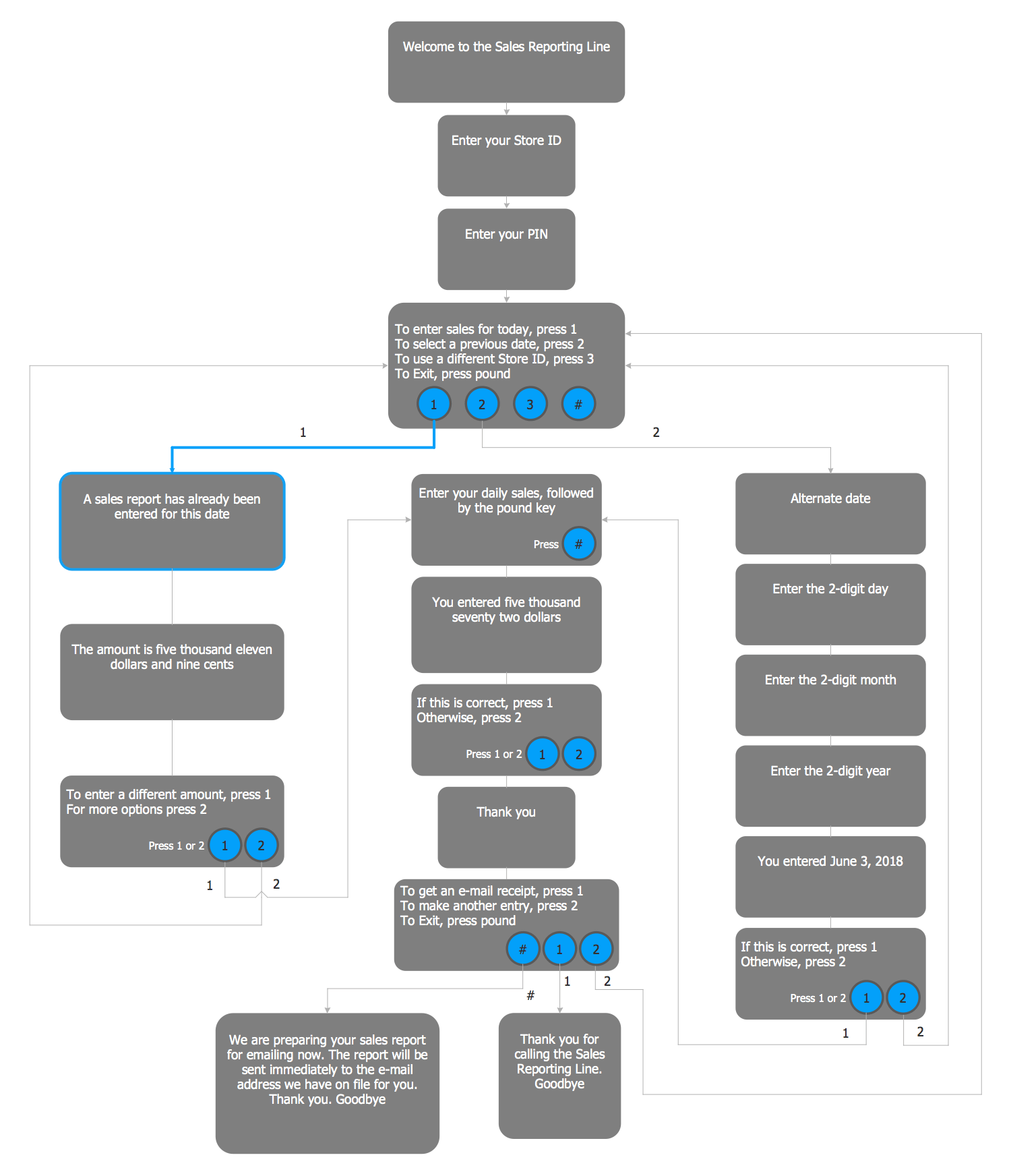## Sample 3: IVR Diagram — Multi-Page

Interactive Voice Response Diagrams sample: IVR Diagram — Multi-Page.

This example is created using ConceptDraw DIAGRAM diagramming and vector drawing software enhanced with Interactive Voice Response Diagrams solution from ConceptDraw Solution Park.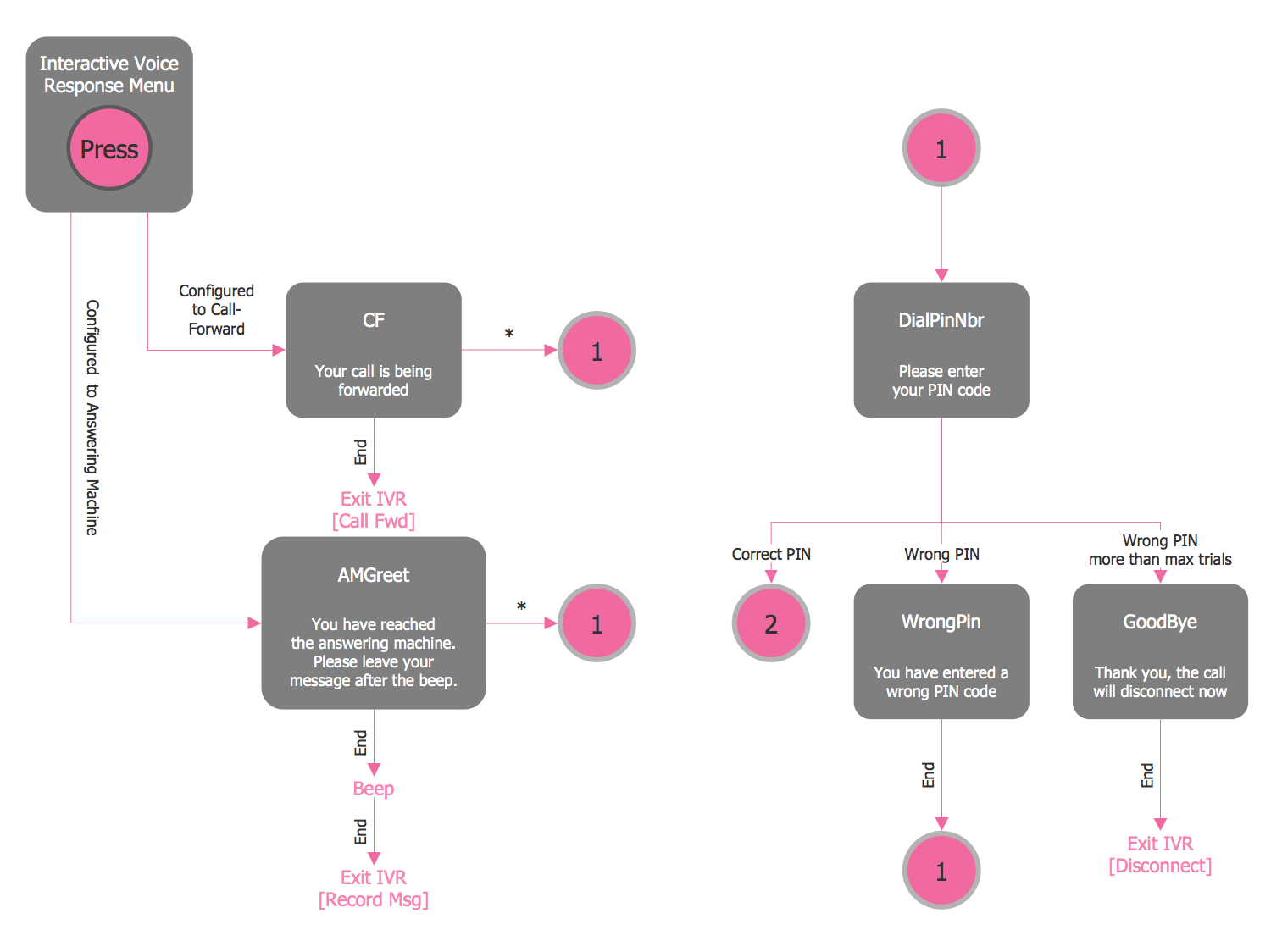## Sample 4: IVR Mobile Operator Diagram

Interactive Voice Response Diagrams sample: IVR Mobile Operator Diagram.

This example is created using ConceptDraw DIAGRAM diagramming and vector drawing software enhanced with Interactive Voice Response Diagrams solution from ConceptDraw Solution Park.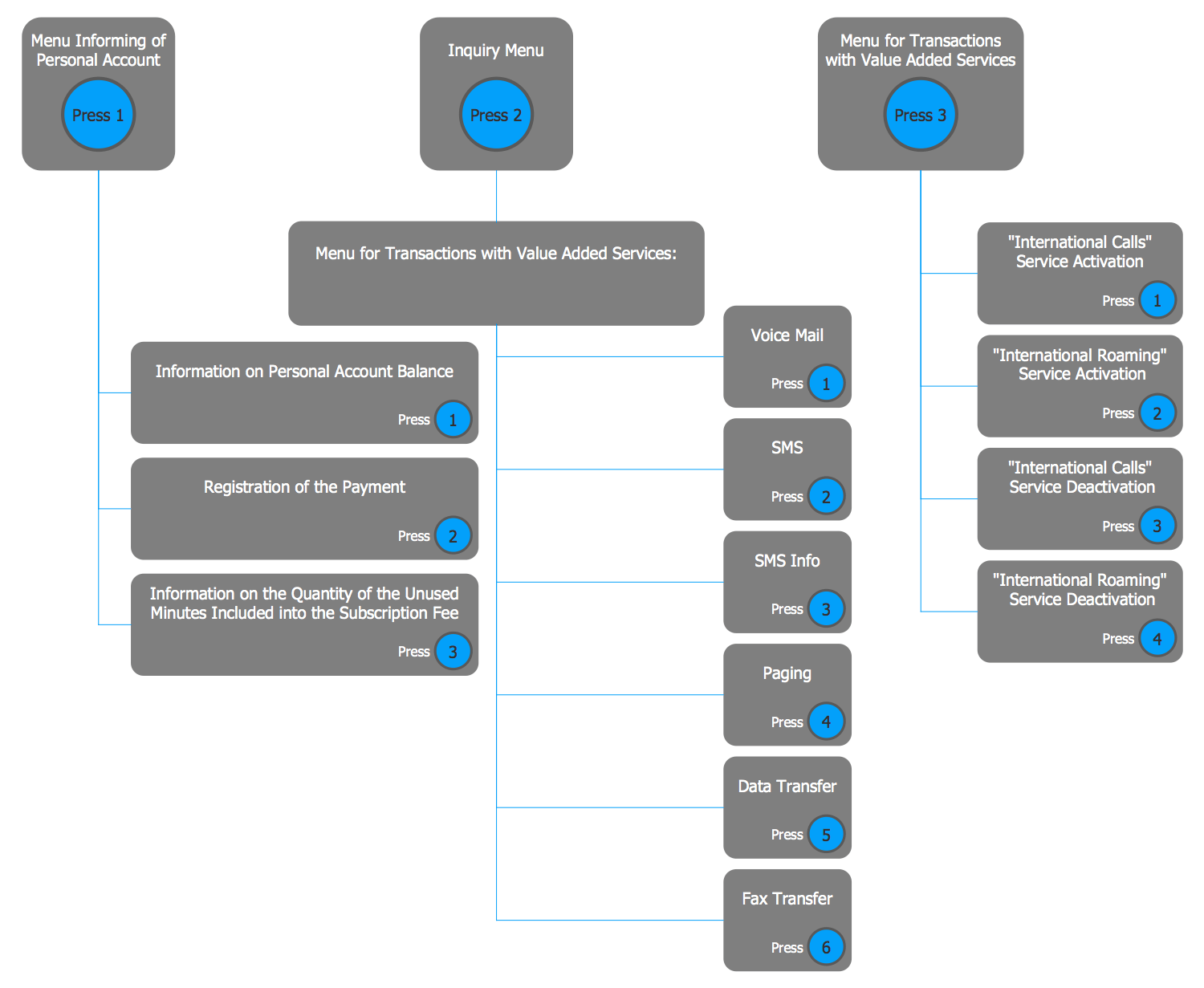## Sample 5: IVR Services Diagram

Interactive Voice Response Diagrams sample: IVR Services Diagram.

This example is created using ConceptDraw DIAGRAM diagramming and vector drawing software enhanced with Interactive Voice Response Diagrams solution from ConceptDraw Solution Park.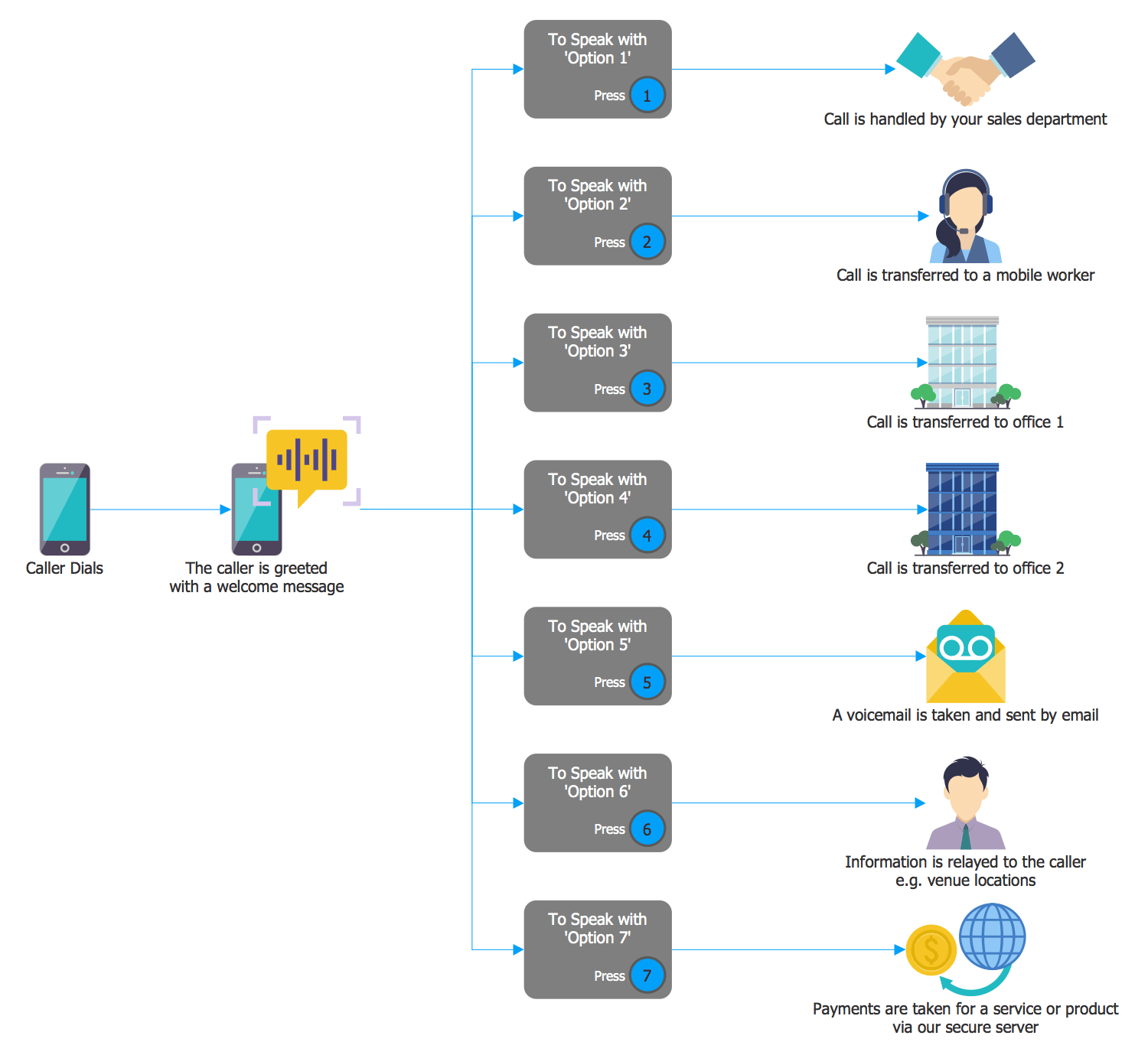## Sample 6: IVR Customer Service Hotline Diagram

Interactive Voice Response Diagrams sample: IVR Customer Service Hotline Diagram.

This example is created using ConceptDraw DIAGRAM diagramming and vector drawing software enhanced with Interactive Voice Response Diagrams solution from ConceptDraw Solution Park.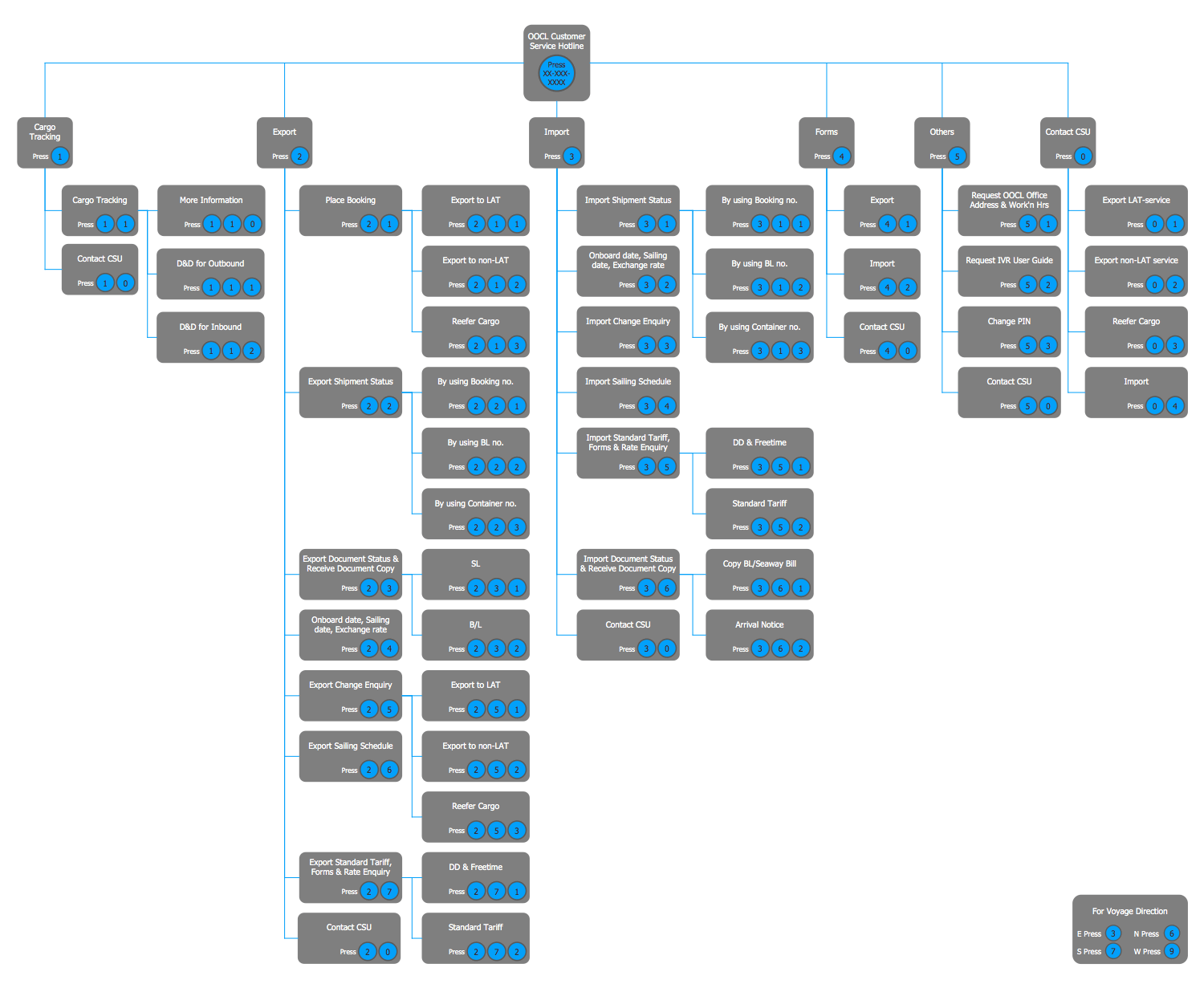## Sample 7: IVR Messaging System Diagram

Interactive Voice Response Diagrams sample: IVR Messaging System Diagram.

This example is created using ConceptDraw DIAGRAM diagramming and vector drawing software enhanced with Interactive Voice Response Diagrams solution from ConceptDraw Solution Park.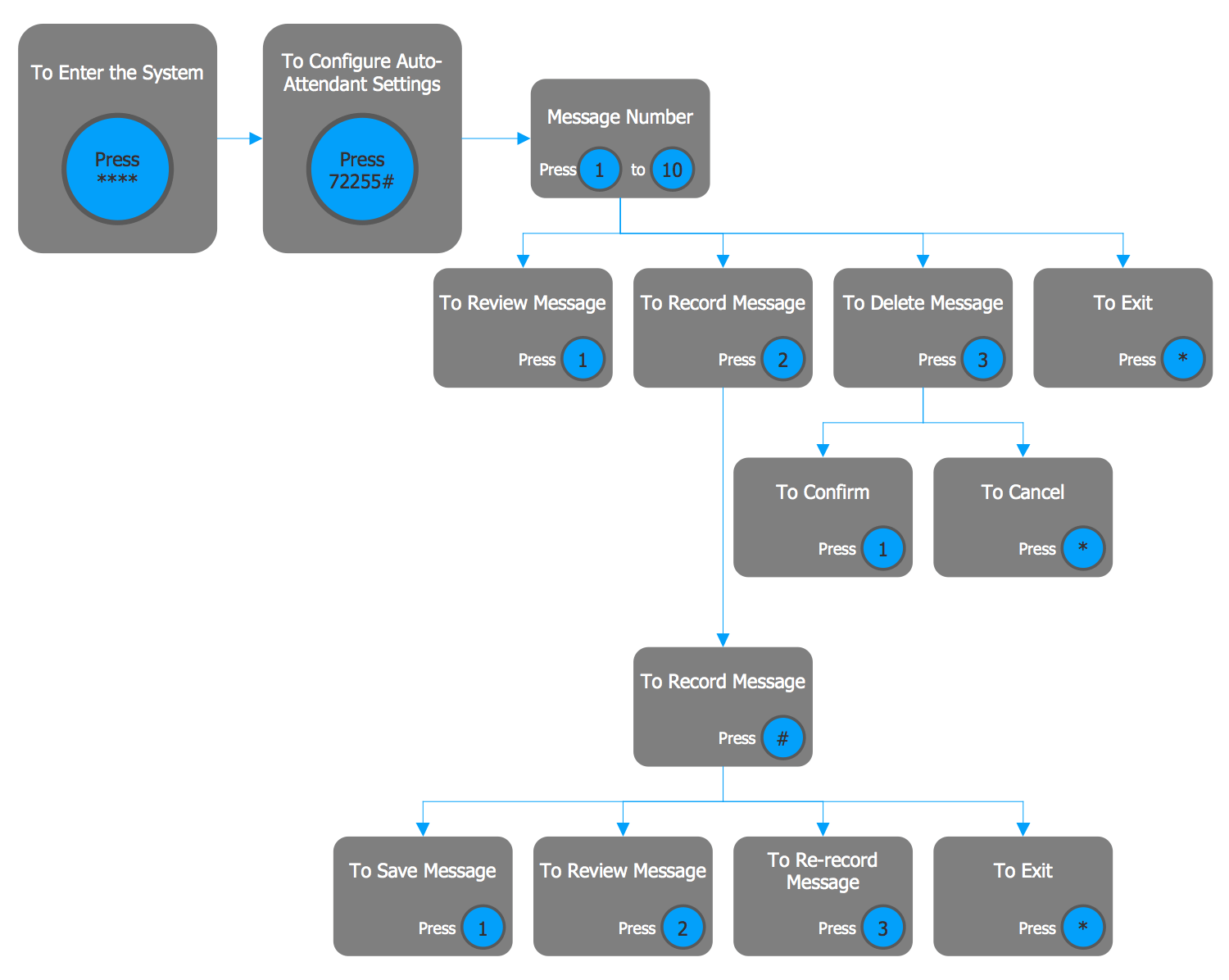## Sample 8: IVR Time Auto-Attendant System

Interactive Voice Response Diagrams sample: IVR Time Auto-Attendant System.

This example is created using ConceptDraw DIAGRAM diagramming and vector drawing software enhanced with Interactive Voice Response Diagrams solution from ConceptDraw Solution Park.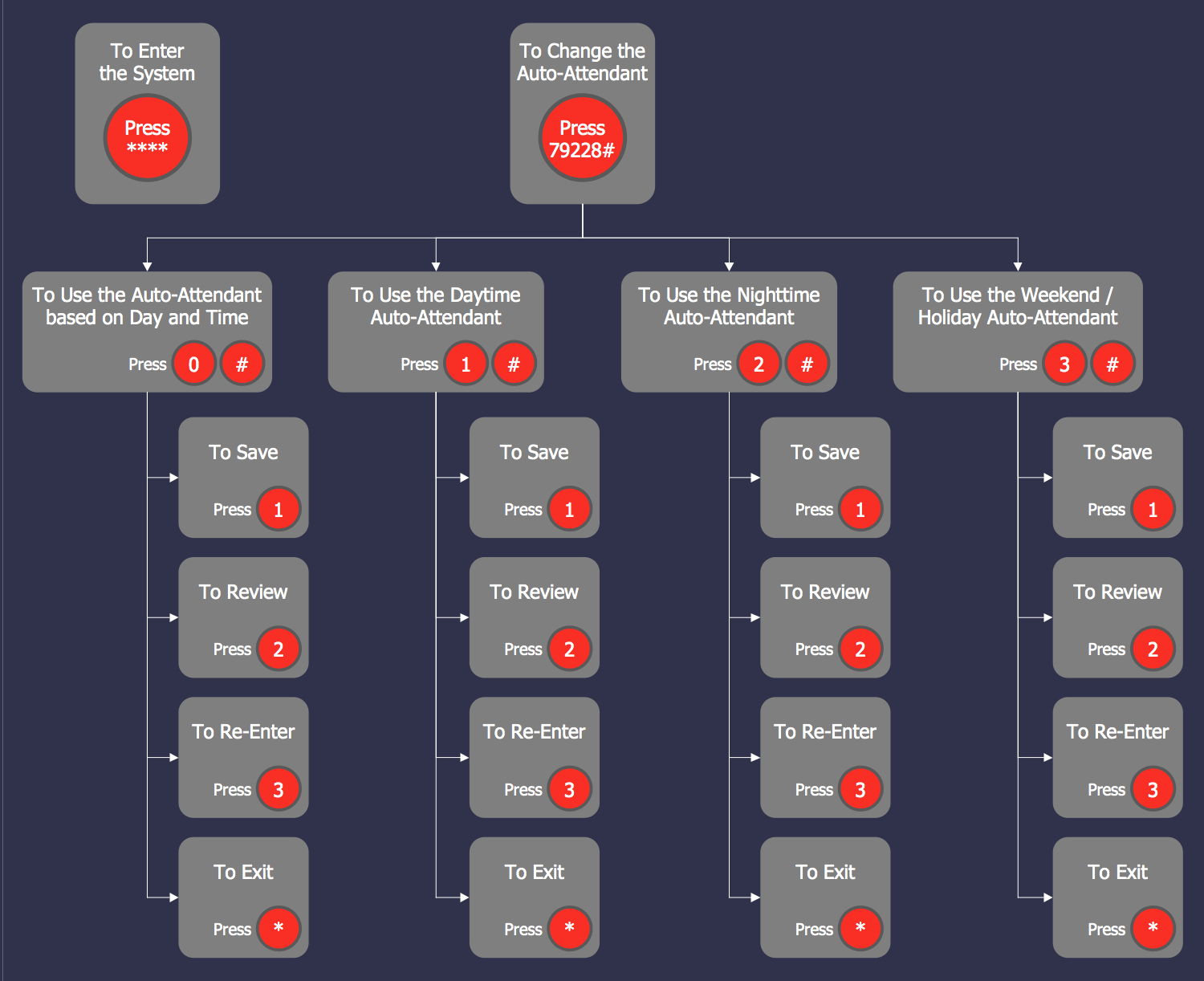## Sample 9: IVR Diagram — IP Telephony Gateway

Interactive Voice Response Diagrams sample: IVR Diagram — IP Telephony Gateway.

This example is created using ConceptDraw DIAGRAM diagramming and vector drawing software enhanced with Interactive Voice Response Diagrams solution from ConceptDraw Solution Park.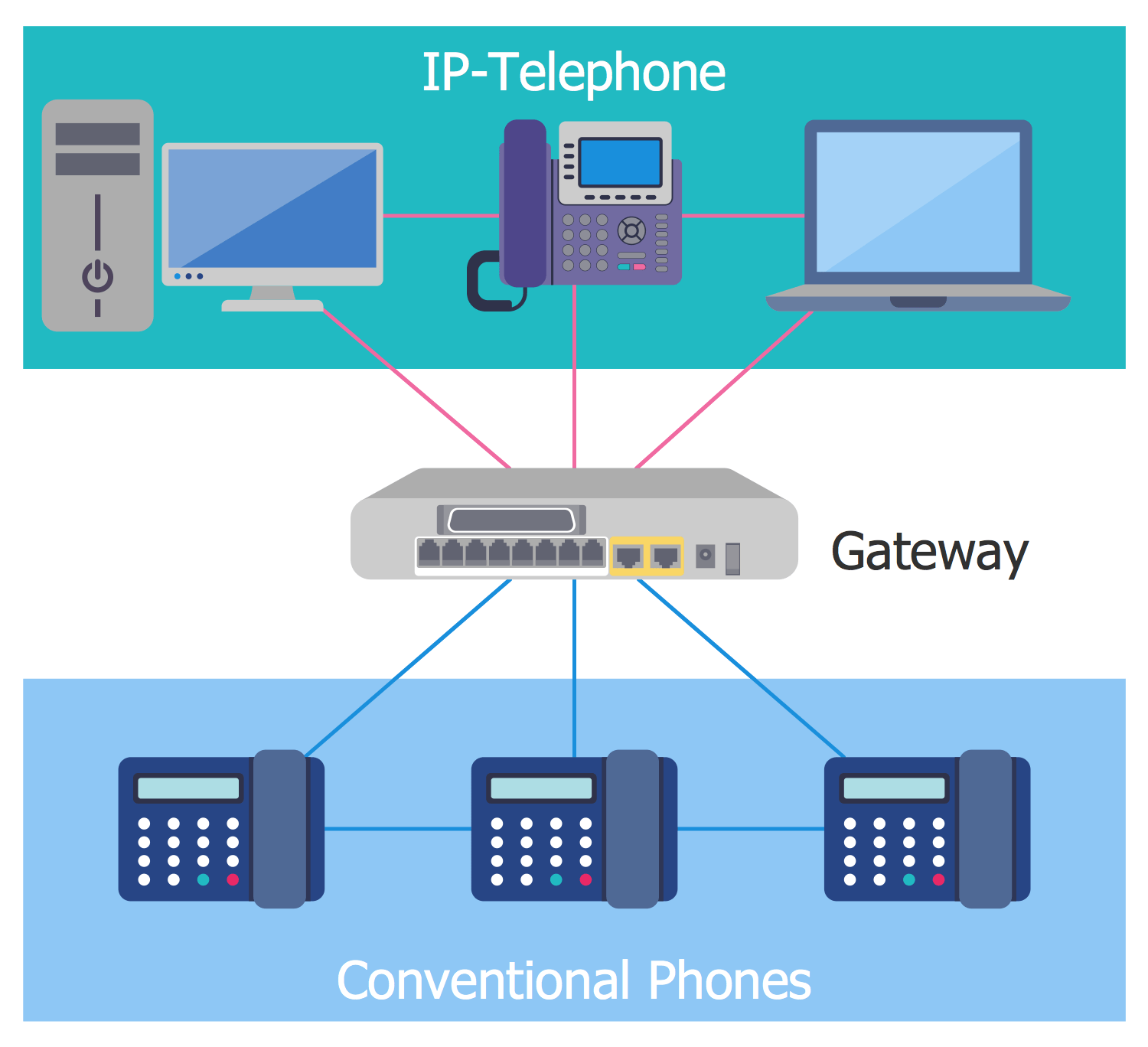## Sample 10: IVR System Architecture

Interactive Voice Response Diagrams sample: IVR System Architecture.

This example is created using ConceptDraw DIAGRAM diagramming and vector drawing software enhanced with Interactive Voice Response Diagrams solution from ConceptDraw Solution Park.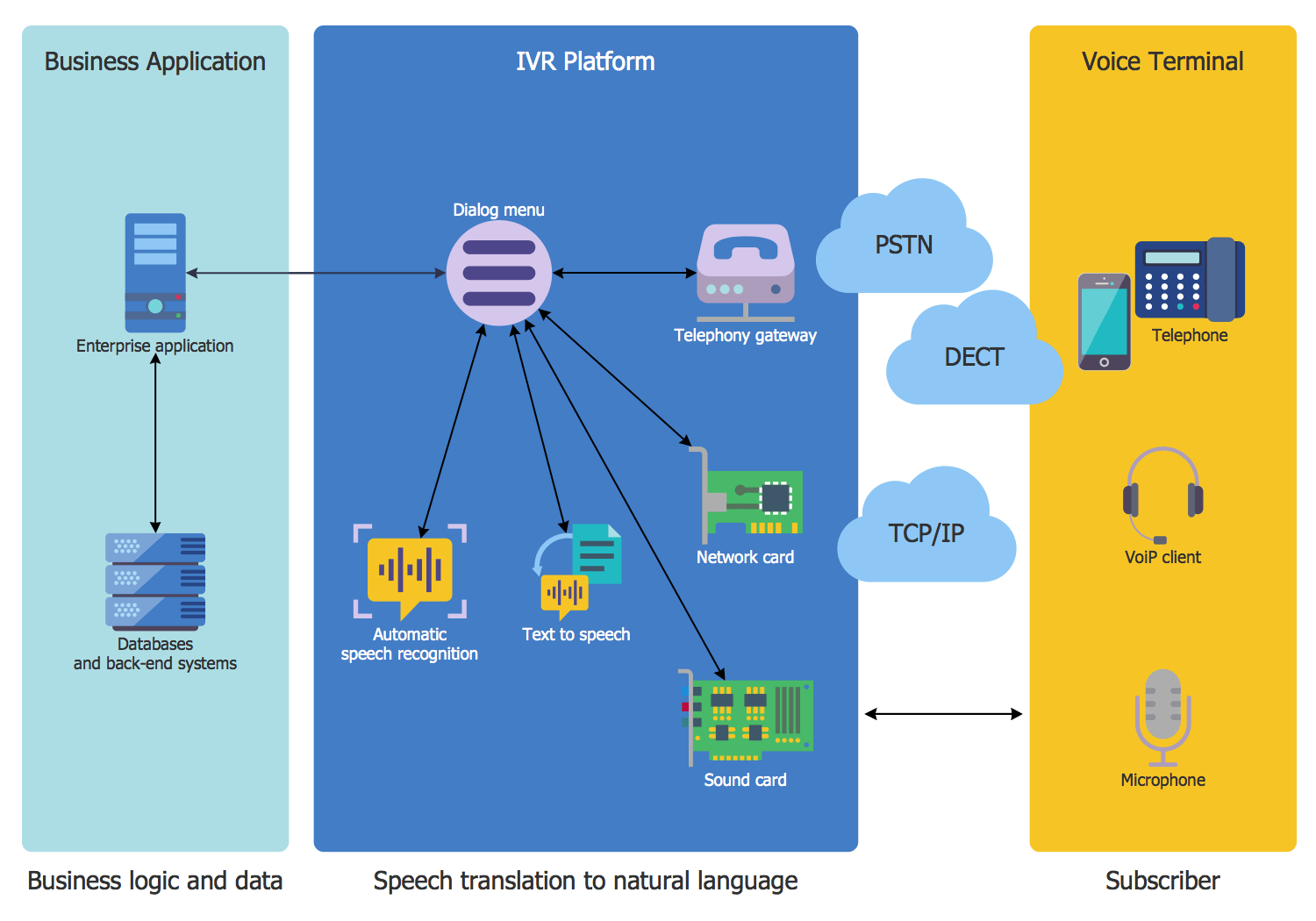## Sample 11: Call Center and IVR

Interactive Voice Response Diagrams sample: Call Center and IVR.

This example is created using ConceptDraw DIAGRAM diagramming and vector drawing software enhanced with Interactive Voice Response Diagrams solution from ConceptDraw Solution Park.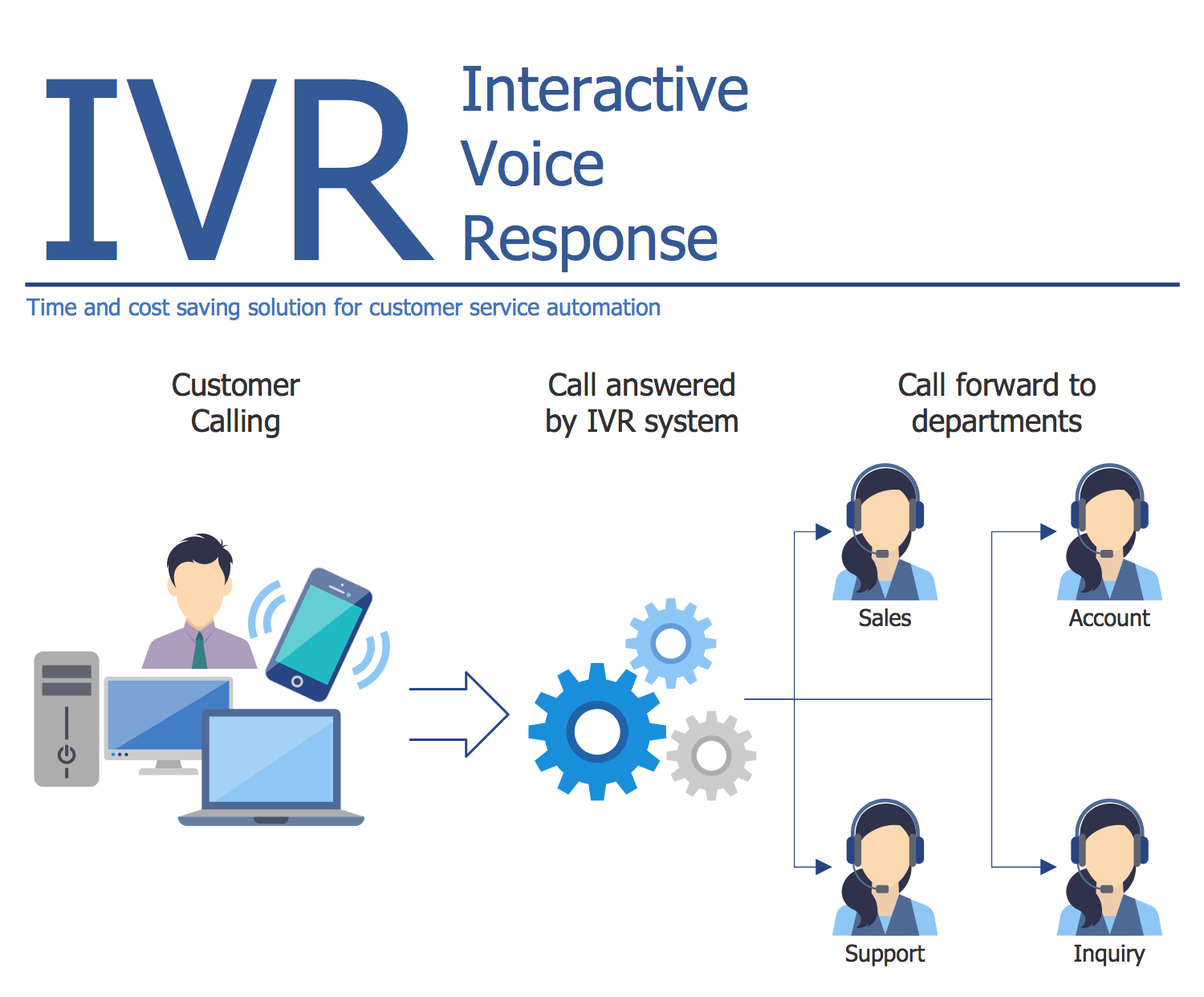## Sample 12: Call Forwarding with ENUM

Interactive Voice Response Diagrams sample: Call Forwarding with ENUM.

This example is created using ConceptDraw DIAGRAM diagramming and vector drawing software enhanced with Interactive Voice Response Diagrams solution from ConceptDraw Solution Park.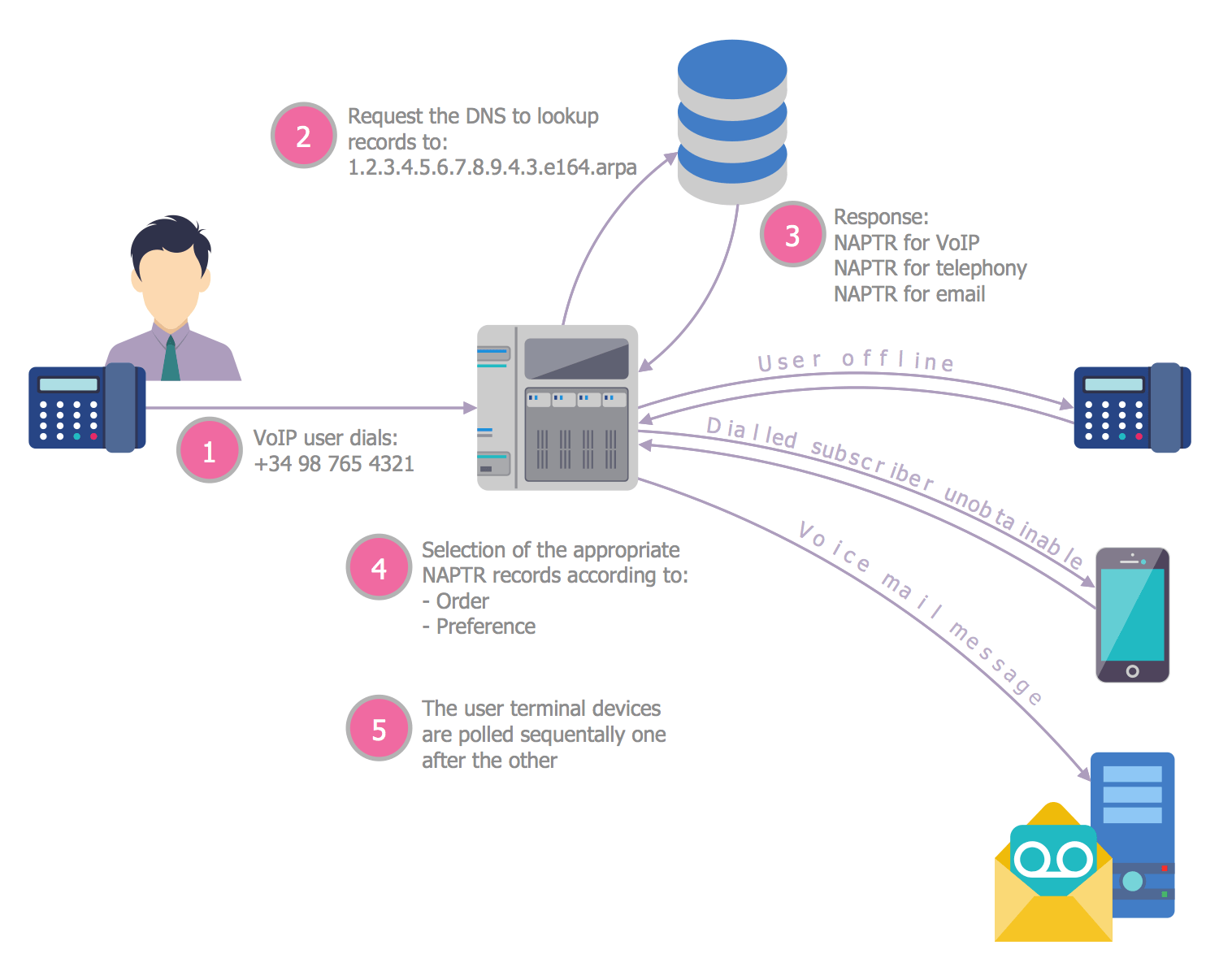## Sample 13: ENUM

Interactive Voice Response Diagrams sample: ENUM.

This example is created using ConceptDraw DIAGRAM diagramming and vector drawing software enhanced with Interactive Voice Response Diagrams solution from ConceptDraw Solution Park.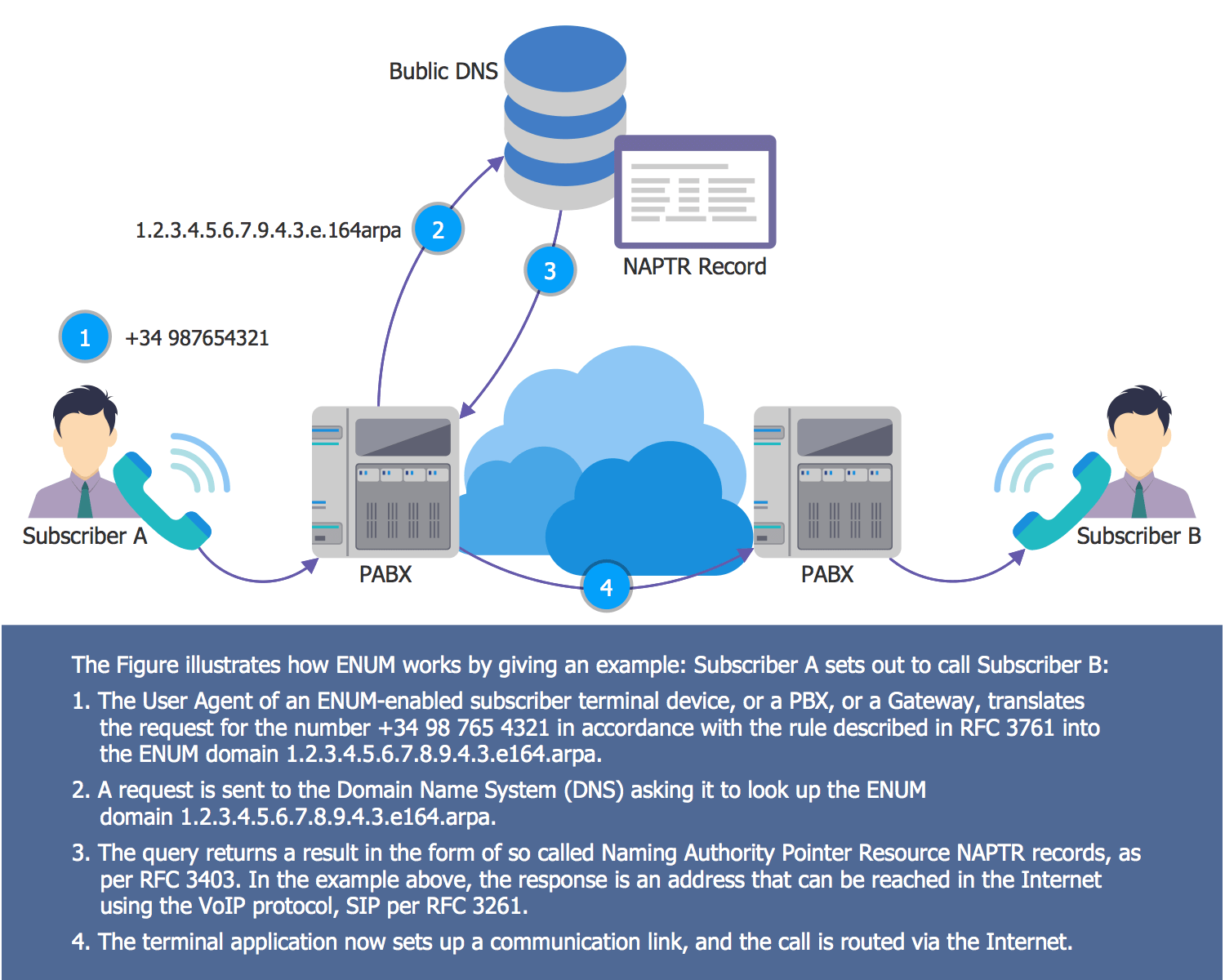## Sample 14: IP Telephony

Interactive Voice Response Diagrams sample: IP Telephony.

This example is created using ConceptDraw DIAGRAM diagramming and vector drawing software enhanced with Interactive Voice Response Diagrams solution from ConceptDraw Solution Park.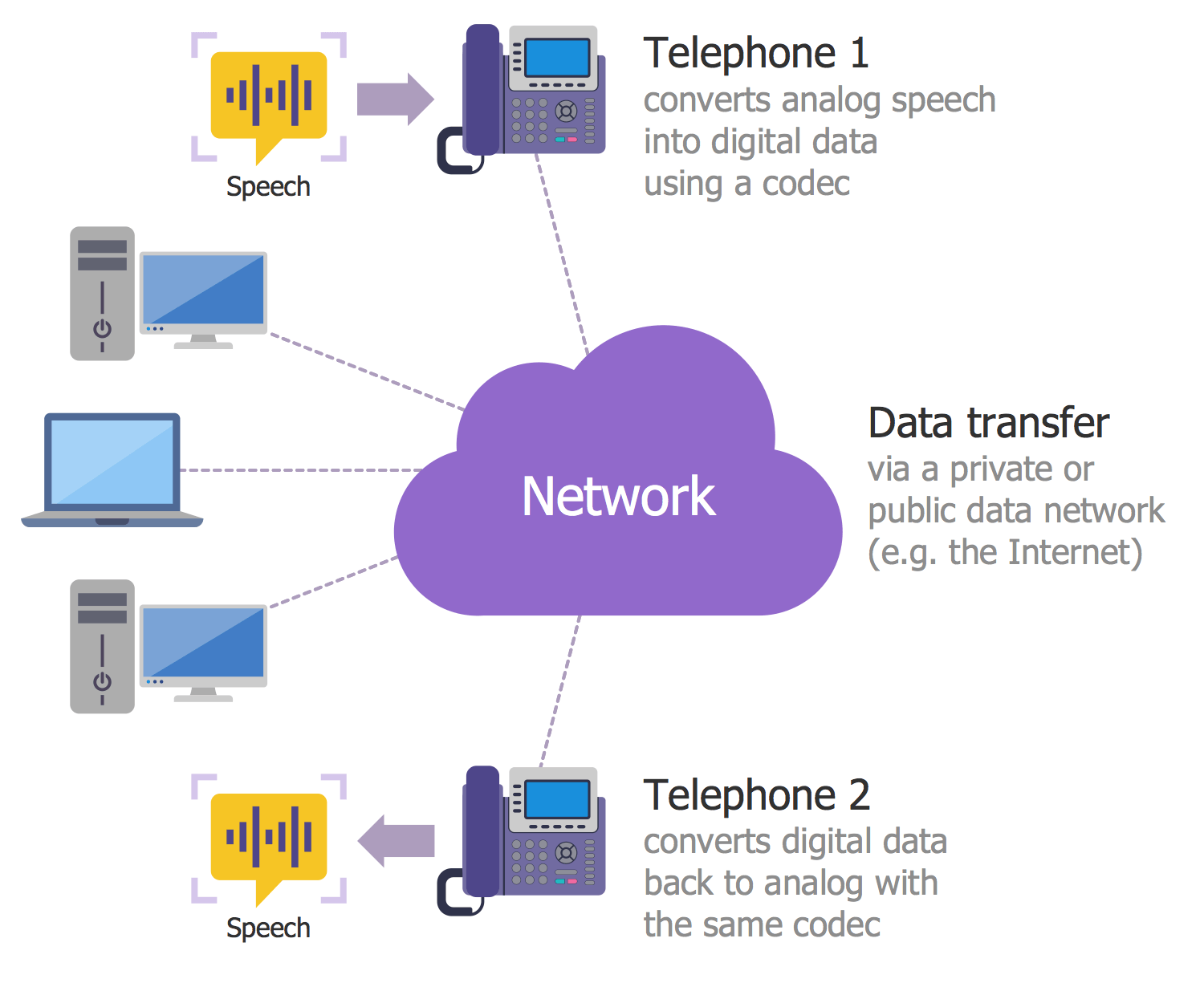## Sample 15: Residential Network Including VoIP

Interactive Voice Response Diagrams sample: Residential Network Including VoIP.

This example is created using ConceptDraw DIAGRAM diagramming and vector drawing software enhanced with Interactive Voice Response Diagrams solution from ConceptDraw Solution Park.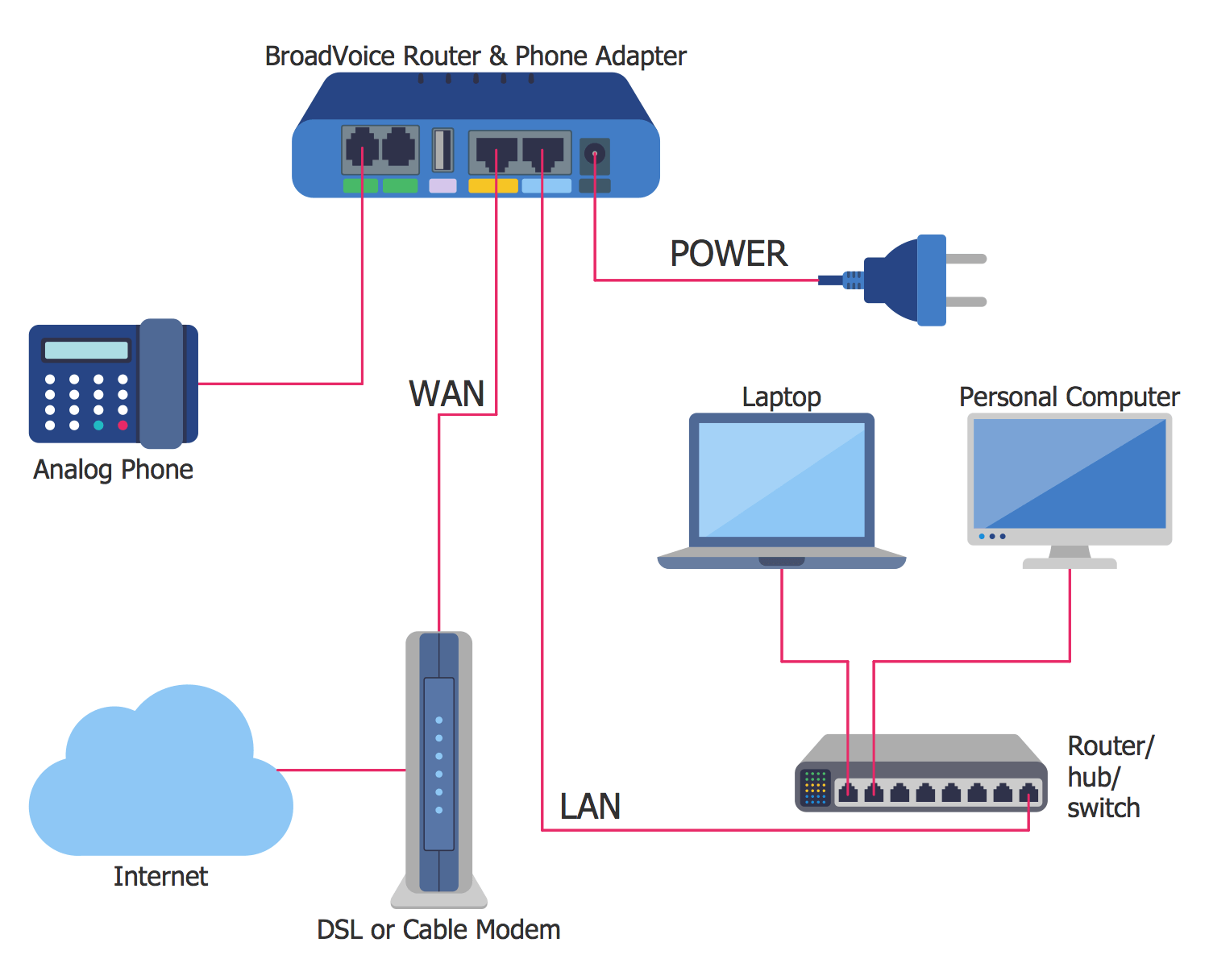## Sample 16: Scheme of VoIP Call with Sim Box

Interactive Voice Response Diagrams sample: Scheme of VoIP Call with Sim Box.

This example is created using ConceptDraw DIAGRAM diagramming and vector drawing software enhanced with Interactive Voice Response Diagrams solution from ConceptDraw Solution Park.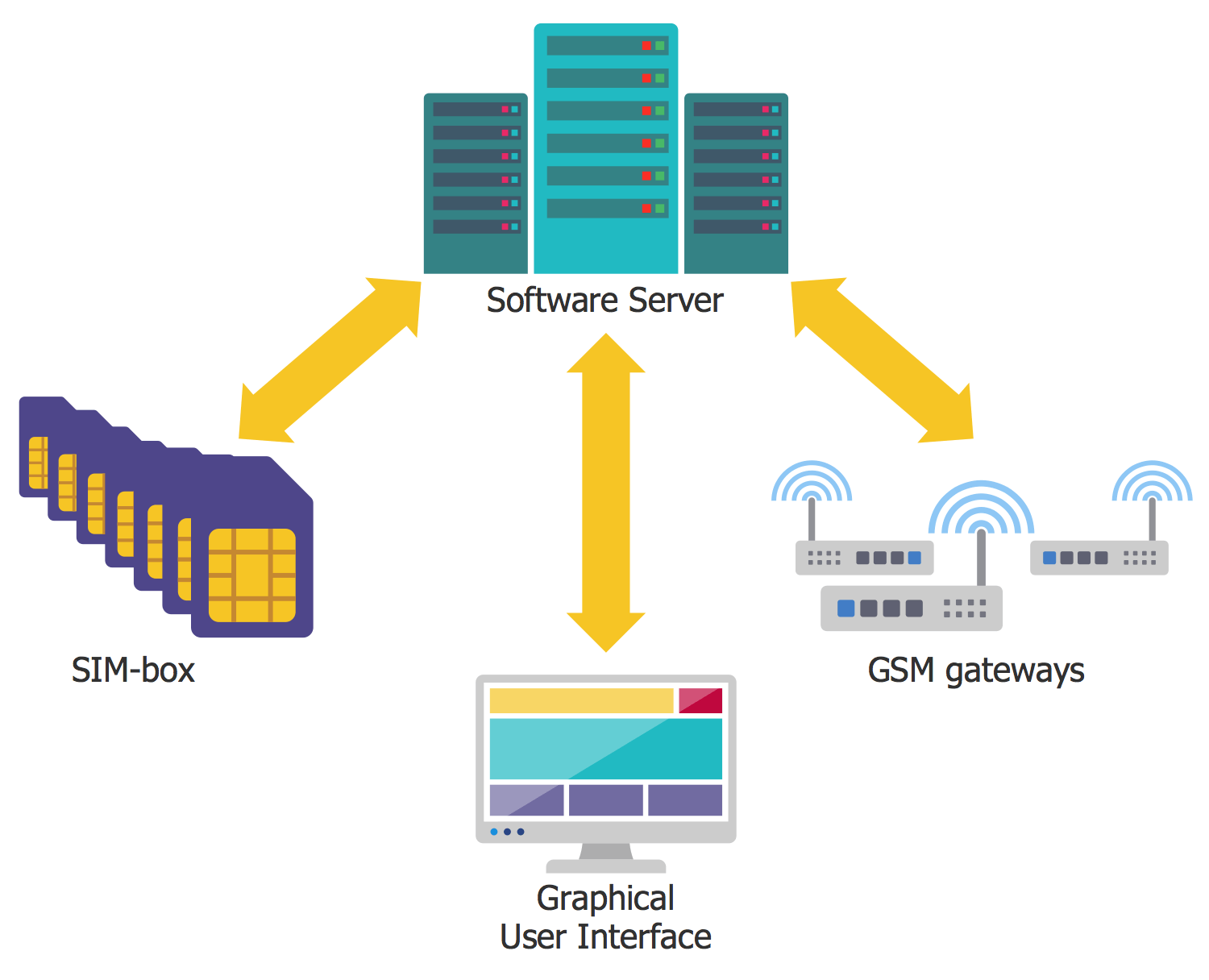## Sample 17: Voice over Internet Protocol

Interactive Voice Response Diagrams sample: Voice over Internet Protocol.

This example is created using ConceptDraw DIAGRAM diagramming and vector drawing software enhanced with Interactive Voice Response Diagrams solution from ConceptDraw Solution Park.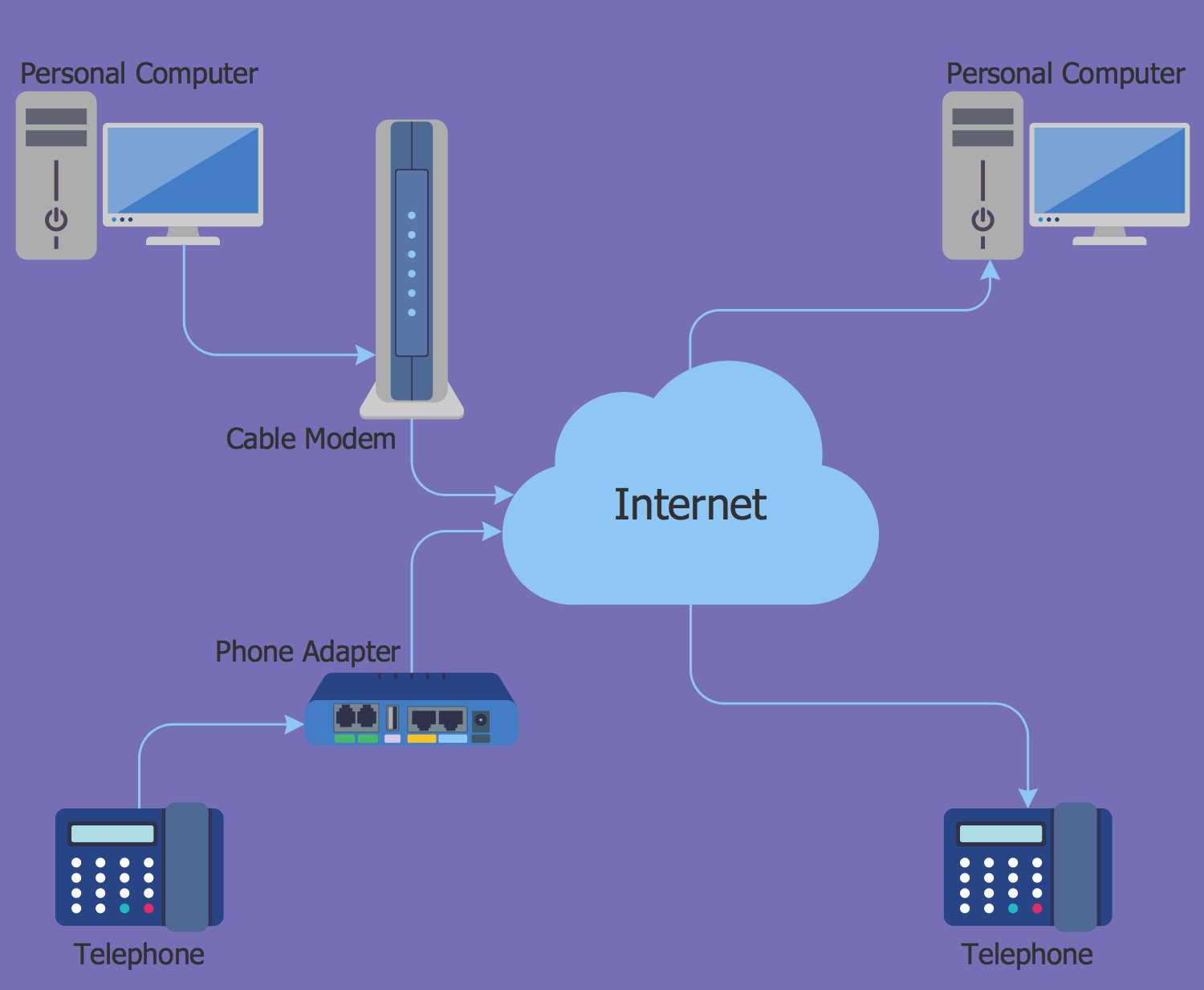## Sample 18: VoIP Interface Architecture

Interactive Voice Response Diagrams sample: VoIP Interface Architecture.

This example is created using ConceptDraw DIAGRAM diagramming and vector drawing software enhanced with Interactive Voice Response Diagrams solution from ConceptDraw Solution Park.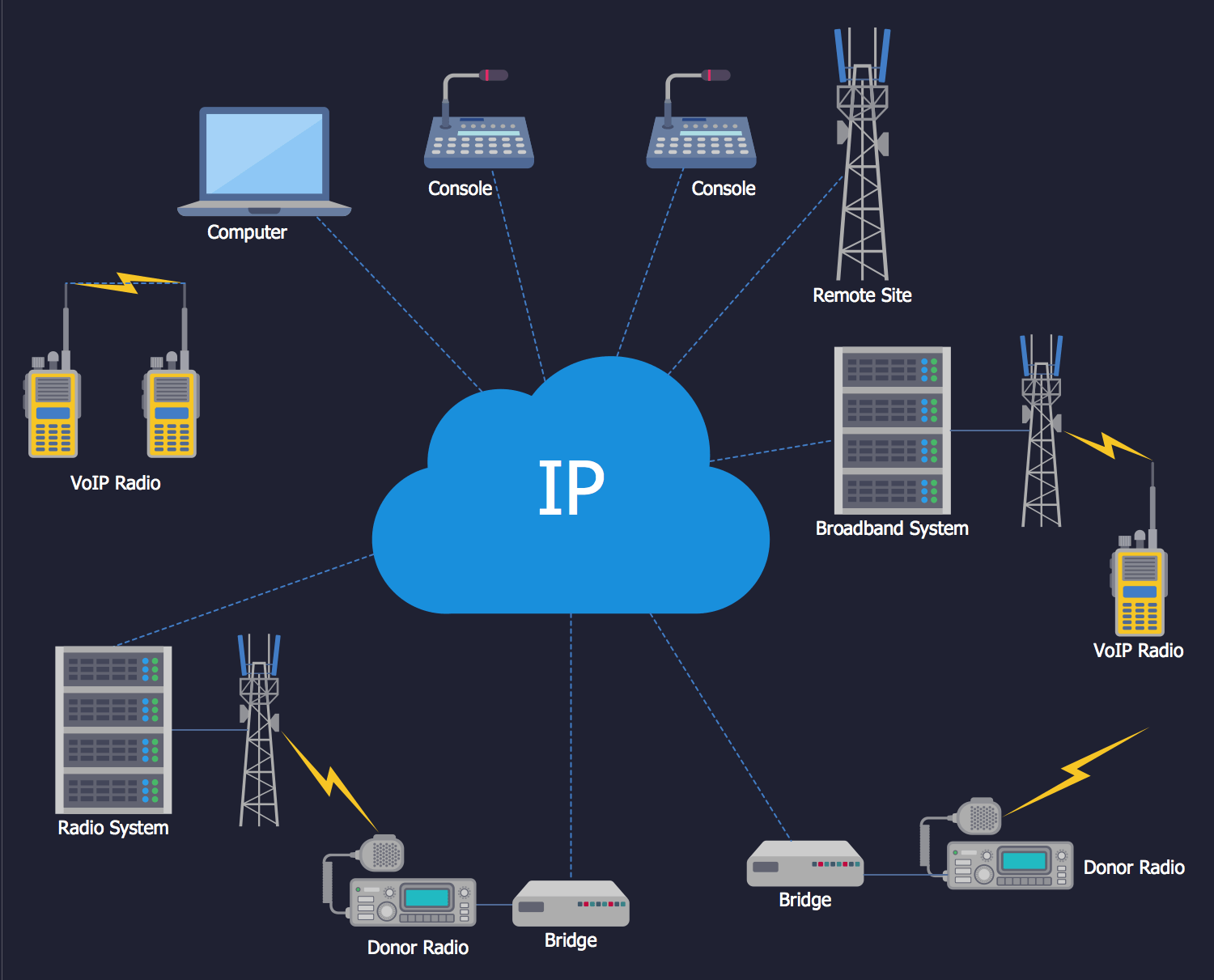## Template 1: Interactive Voice Response Template

Interactive Voice Response Diagrams template: Interactive Voice Response Template.

This example is created using ConceptDraw DIAGRAM diagramming and vector drawing software enhanced with Interactive Voice Response Diagrams solution from ConceptDraw Solution Park.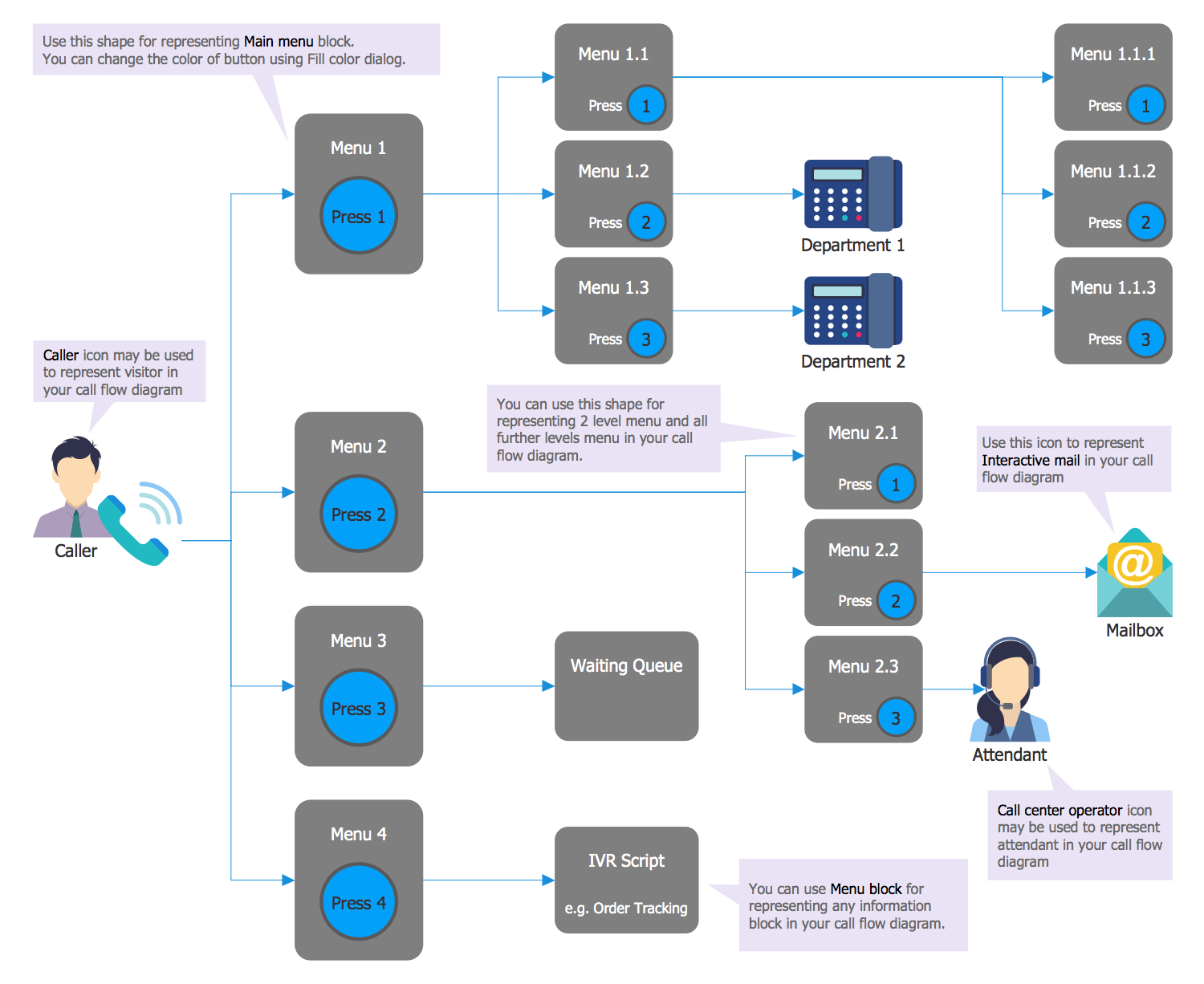All samples are copyrighted CS Odessa's.

Usage of them is covered by Creative Commons “Attribution Non-Commercial No Derivatives” License.

The text you can find at: https://creativecommons.org/licenses/by-nc-nd/3.0